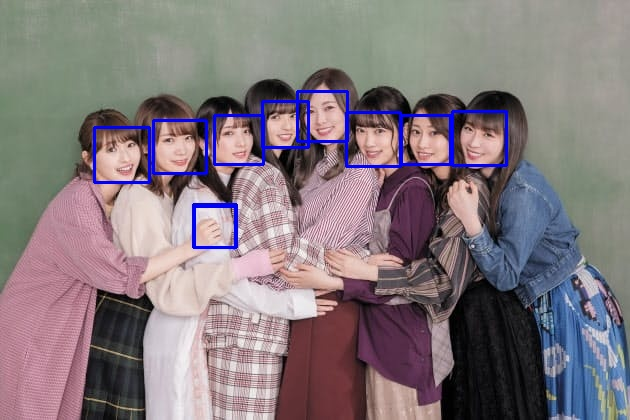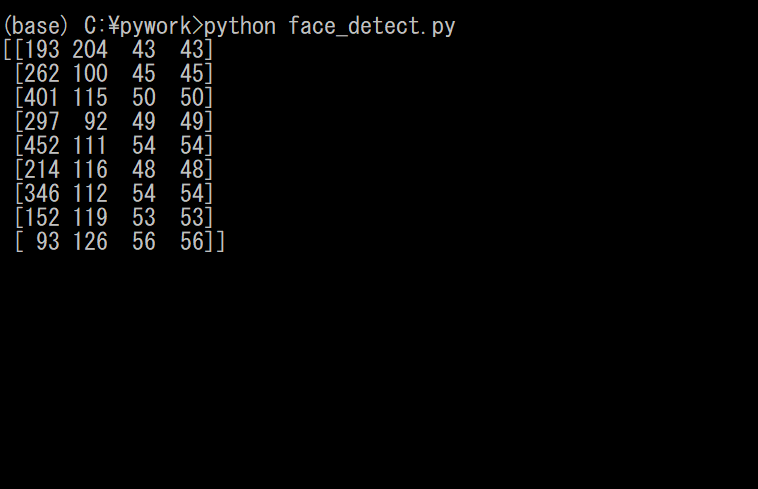# 1. Background

Currently, I am writing a program that recognizes faces using deep learning. First, a large number of face images were prepared as learning data. At this time, the face was detected from the image showing the person, and only the face part was cut out. Of these, a cascade classifier was used as a method for detecting faces. This article describes a cascade classifier.

# 2. What is a cascade classifier?

A cascade classifier is a classifier composed of multiple simple classifiers. These simple classifiers are applied in sequence to the target image. It is detected after passing through all the classifiers. On the other hand, if it is rejected even once by a simple classifier, it will not be detected at that point. Therefore, images that clearly do not correspond to the detection target are rejected at the initial stage, which is fast. In OpenCV, a cascade classifier using HAAR-Like features is prepared.

The HAAR-Like feature is a feature based on the brightness and darkness of the image. It is characterized by the difference between the white area and the black area in the local area of the image. Therefore, it is common to convert the image to grayscale before using it.

# 3. Cascade classifier in OpenCV

In OpenCV, after reading the cascade file containing the cascade classifier, it is detected by detect.MultiScake (). The return value of detect.MultiScake () is an N × 4 matrix, where N is the detected number and 4 is the 4 elements of [x, y, width, height]. These four elements are the areas where the detected area is surrounded by a rectangle. x is the upper left x coordinate of the rectangle, y is the upper left y coordinate of the rectangle, width is the width of the rectangle, and height is the height of the rectangle. Therefore, if you want to mark the detected part, use the OpenCV drawing function. You can write rectangle (image, (x, y), (x + width, y + height), (255,0,0), 2) etc. using rectangle (). Try it on the image below.The source code is shown below.

#### `face_detect.py`

``````
import cv2

#Convert image to grayscale
image_gray = cv2.cvtColor(image, cv2.COLOR_RGB2GRAY)

#Read a classifier from a file

#Detect using a classifier
faces = cascade.detectMultiScale(image_gray, scaleFactor=1.1, minNeighbors=1, minSize=(1, 1))

#detectMultiScale()Output the return value of(For confirmation)
print(faces)

#Mark the part of the detected face image with a rectangle
for (x,y,width,height) in faces:
cv2.rectangle(image,(x,y),(x+width,y+height),(255,0,0),2)

#Display image
cv2.imshow('result',image)
cv2.waitKey(0)
cv2.destroyAllWindows()
``````

The execution result is as follows. There is only one misrecognition, but all faces can be detected.By the way, since the return value of detectMultiScale () was output, if you look at the output result ...As shown above, the information of the upper left coordinates, width, and height in the detected area (rectangle) is returned.

# 4. Digression

In the previous article, I learned how to cut out only a part of an image using the array returned by imread.